# CONCEPTS OF SETS IN PYTHON PROGRAMMING

in STEMGeeks5 months agoSource

Set is basically one of major 4 built-in data types in python that is used to store the collection of objects. Sets are mutable data types and you can use it when you don't want the items to be stored in a particular order or when you don't want the duplicate items. Since, there's no any definite order in which items are stored, indexing has no use here as you do with list for accessing and modifying. However python allows you to add new value to your existing set. In order to define a set, you need to use curly braces`{}` as you do with mathematical sets.

Lets see a practical example on this one. Create a new python file, named it anything you like and lets run this simple code for now:

``````my_set = {1, 2, 3, 4, 5}
print(my_set)
``````

If you run this code, you will see this output: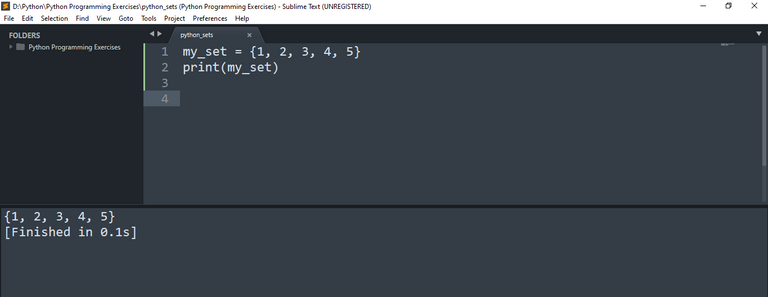Here you maybe thinking that our program prints the set in the same order as we have defined in `my_set` variable but this is actually not the case. Lets define another similar type of set and print it.

``````my_set = {1, 2, 3, 4, 5}
print(my_set)

another_set = {6, 7, 8, 9}
print(another_set)
``````

Now you can see this output: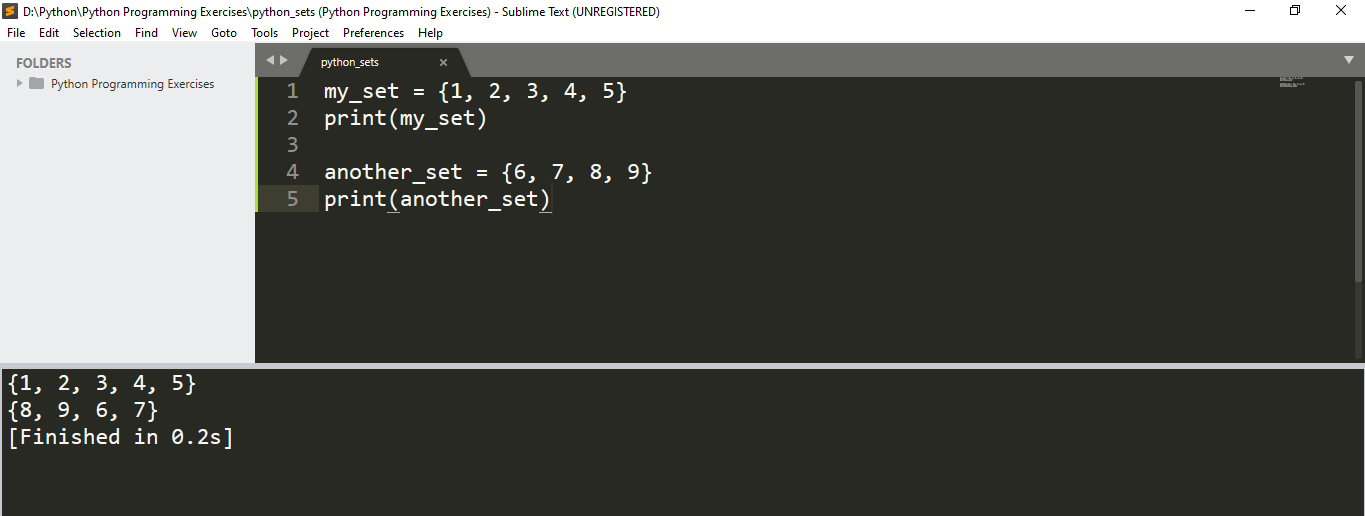The first set was printed in the same order as we have defined but not the second one. Its just a random order in which it gets printed.

If you try modifying its third value (i.e. index number 2) by writing `my_set = 3.5` to above code, you will get this error: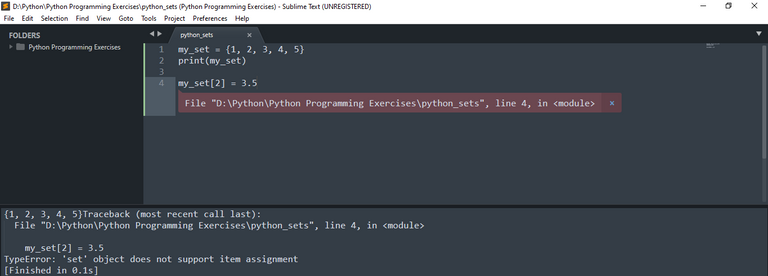You can find the length of this set using `len()` function:

``````my_set = {1, 2, 3, 4, 5}
print(my_set)

print("The length of the above set is", len(my_set))
``````

Here's the output: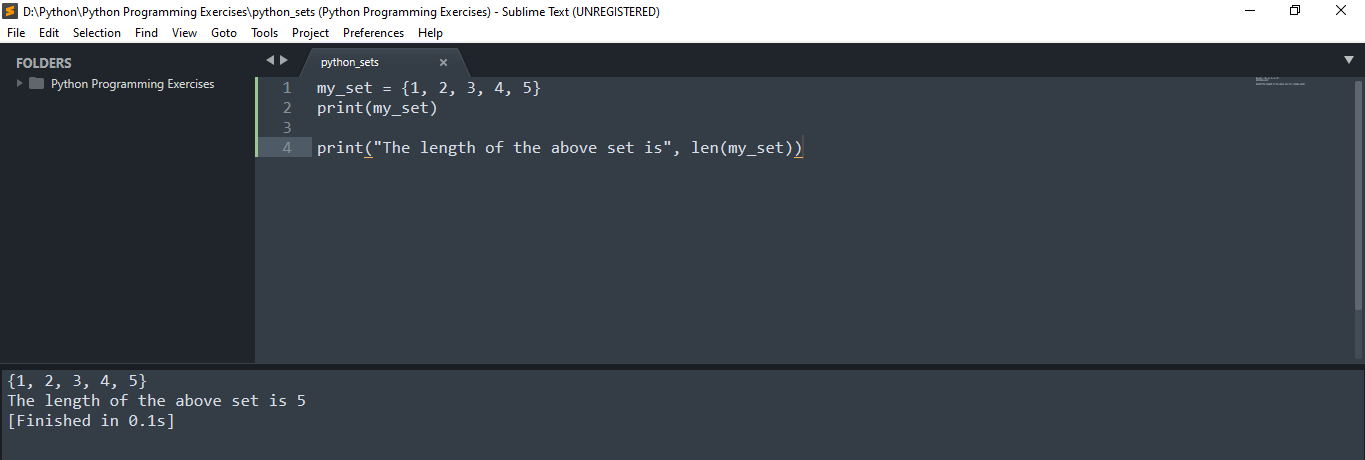Now lets see on how you can add a new element to your set. It is simple to do this just by using `add()` function. See this code for your reference:

``````my_set = {1, 2, 3, 4, 5}
print("The original set is", my_set)

print("The set after addition is", my_set)
``````

If you run this code, then you will get the following output: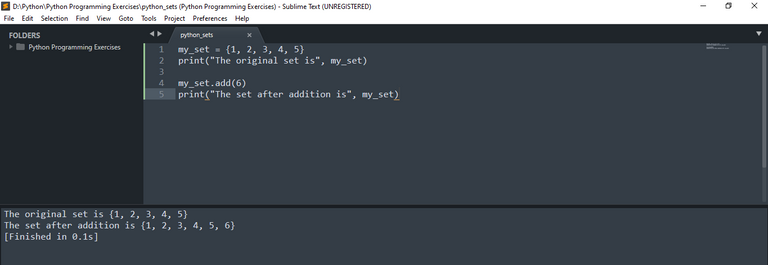You can see 6 has been added to our original set. Instead of using `my_set.add(6)` lets see by writing `my_set.add(3)` to the above code to see that set doesn't allow storing of duplicate values. You can see the following output.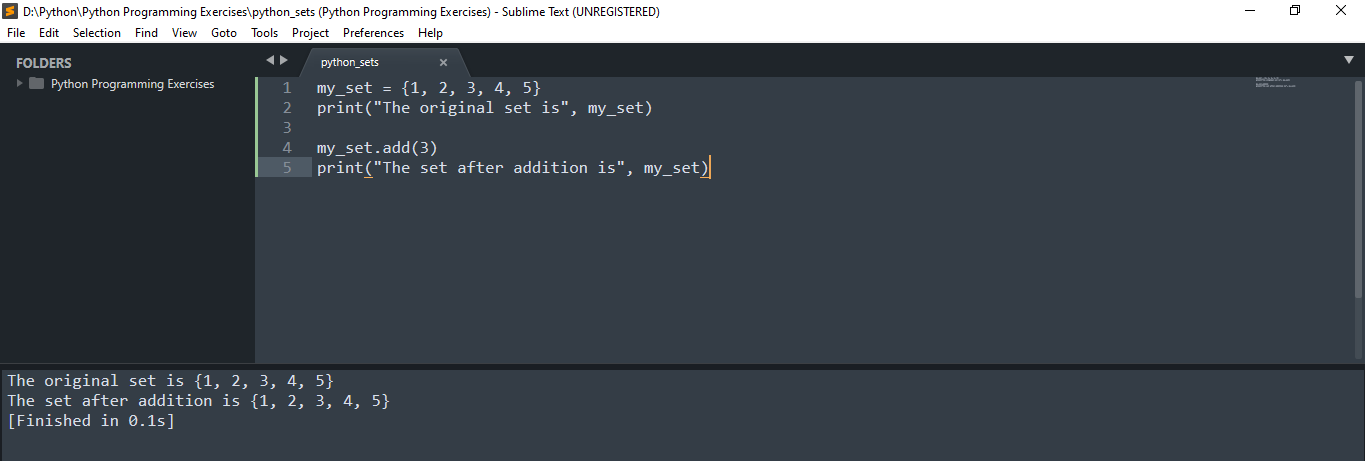But the disadvantage of this function is that you can add only one value to your exisiting set. You can't add two or more values. For example, see this picture below where I tried to add two values and it returned error as `add()` function takes only one parameter but 2 were given.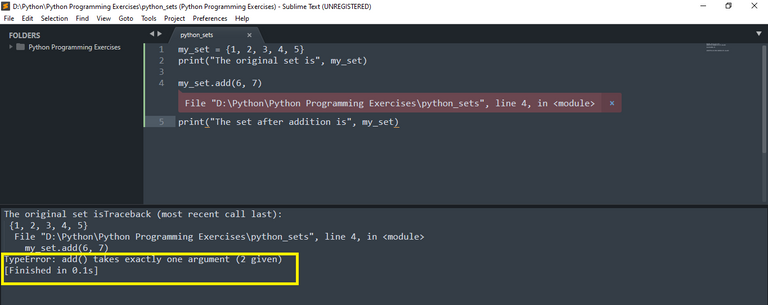To remove this drawbacks, we can use `update()` function. It either takes list, set itself, tuple or dictionary as arguments and add to our original set.

``````my_set = {1, 2, 3, 4, 5}
print("The original set is", my_set)
print()
my_set.update({6, 7, 8, 9})
print("The set after addition is", my_set)
``````

If you execute this code, you can see those value added to your original set.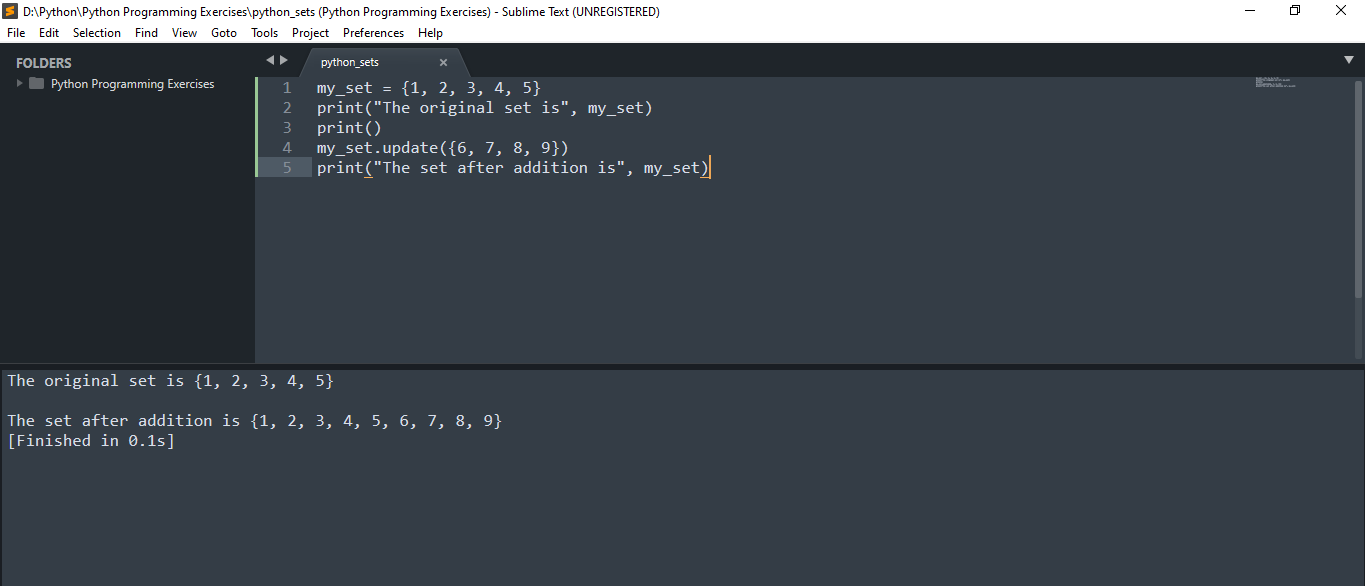Another simple approach to this could be using for-loop or while loop. But this process is tedious as it is long but simple. This can you help just for learning programming. The program below will ask user how many value they want to add to the set. Then it will use a loop prompting user to enter the values for specified number. Then it will use `add()` function to add the value to the original set for each iteration. And after the loop exits, we print out the original set with added items.

``````my_set = {1, 2, 3, 4, 5}
print("The original set is", my_set)
print()

count = int(input("Enter how many values you want to add to set: "))

for i in range(1, count+1):

new_item = input("Enter value to add to the set: ")

print("The new set is: ", my_set)
``````

Let me show you the demo for this: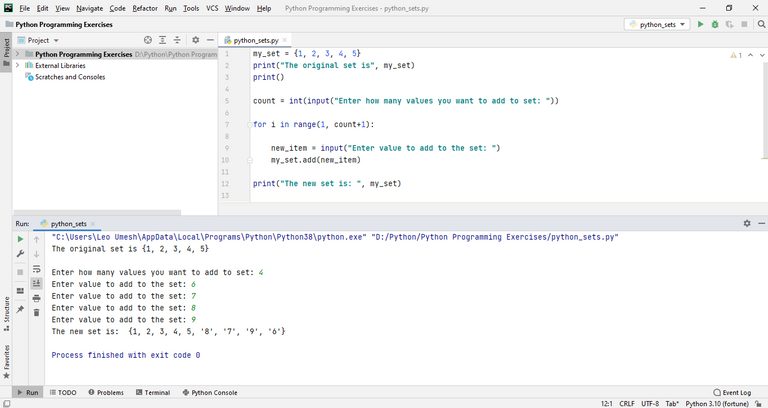You can not only add numerical values to this set but also character values. Here is the demo illustrating the same concepts.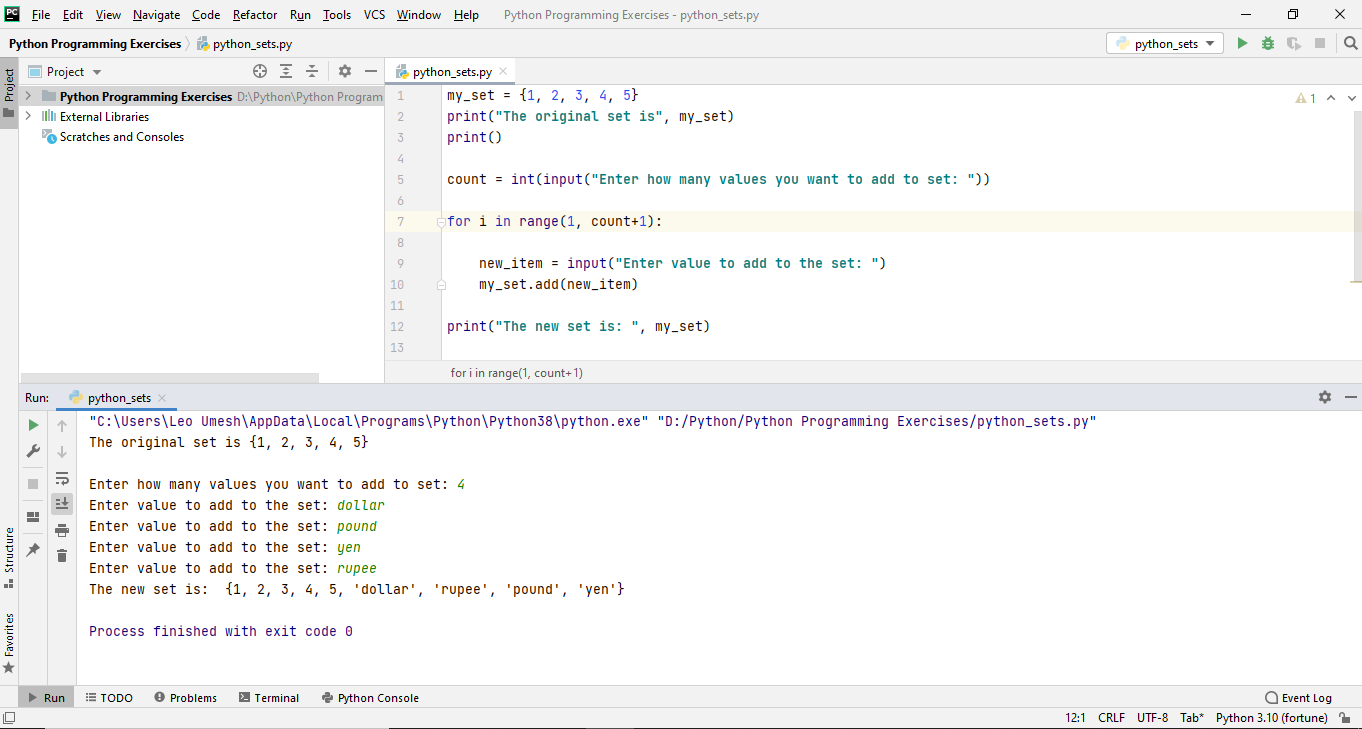I have to use PyCharm community edition for this one. Because my Sublime Text was unregistered and I couldn't run the loop because Unregistered Sublime Text won't allow you to use feature of interactive console like allowing user to enter the input. So that's why I switch to PyCharm to execute this code. Using Sublime Text is friendly and lightweight.

In my next post, I will write on applying different operations to set like union, intersection and also different ways of removing the items from set. You can try running this code online in this website.